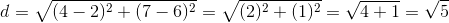# SAT II Math II : Distance Formula

## Example Questions

### Example Question #1 : Distance Formula

What is the distance between the point (1,2) and (8,5)?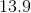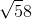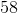Explanation:

For this question we will use the distance formula to solve.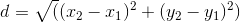In our case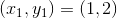and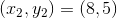Substituting these values in we get the following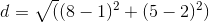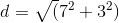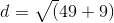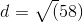### Example Question #2 : Distance Formula

The points A=(-2,0), B=(0,3), and C=(0,0) makes a triangle.  What is the distance between point A and point B?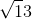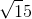Explanation:

For this question we need to use the distance formula for points A and B.

Point A will be our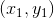and point B will be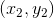Now we substitute these values into the following: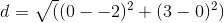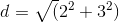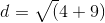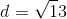### Example Question #3 : Distance Formula

Find the distance between the two points (2,7) and (4,6).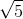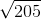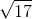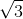Explanation:

The distance between two points is found using the formula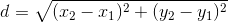For this problem the values are as follows: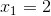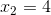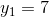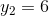Input the values into the formula and simplify